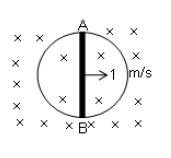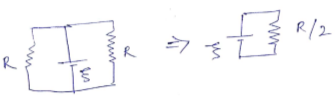#### A circular loop of radius 1m is kept in a magnetic field of strength 2 T directed perpendicular to the plane of loop. Resistance of the loop wire is 2/π Ω/m. A conductor of length 2 m is sliding with a speed 1 m/s as shown in the figure. Find the instantaneous force acting on the rod [Assume that the rod has negligible resistance]Option 1) 8 N Option 2) 16 N Option 3) 32 N Option 4) 64 N

Induced Current --

Given figure can be redrawn as:Force on wire = I. l.BOption 1)

8 N

Incorrect

Option 2)

16 N

Correct

Option 3)

32 N

Incorrect

Option 4)

64 N

Incorrect